# 第三章 深度学习：Pytorch的使用

## Pytorch库基础

### Tensor 张量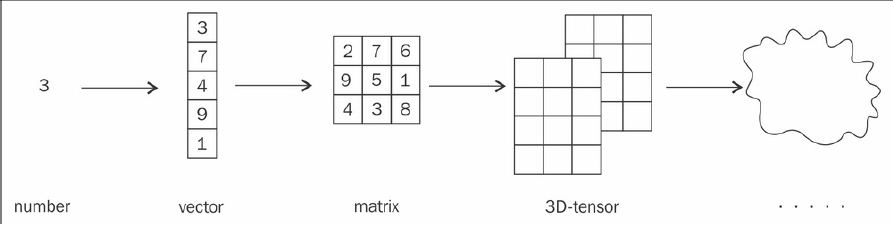• 直接调用 对应类型张量的构造器
>>> import torch
>>> a = torch.FloatTensor(3, 2)
>>> a
tensor([[ 4.1521e+09, 4.5796e-41],
[ 1.9949e-20, 3.0774e-41],
[ 4.4842e-44, 0.0000e+00]])


>>> a.zero_()
tensor([[ 0., 0.],
[ 0., 0.],
[ 0., 0.]])


• 另一种创建方式 是 转换 python中的 可迭代对象， 如 list, tuple, numpy数组等， 将其作为张量的内容来创建张量。
>>> torch.FloatTensor([[1,2,3],[3,2,1]])
tensor([[ 1., 2., 3.],
[ 3., 2., 1.]])
>>> import numpy as np
>>> n = np.zeros(shape=(3, 2))
>>> n
array([[ 0., 0.],
[ 0., 0.],
[ 0., 0.]])
>>> b = torch.tensor(n)
>>> b
tensor([[ 0., 0.],
[ 0., 0.],
[ 0., 0.]], dtype=torch.float64)


>>> n = np.zeros(shape=(3, 2), dtype=np.float32)
>>> torch.tensor(n)
tensor([[ 0., 0.],
[ 0., 0.],
[ 0., 0.]])


>>> n = np.zeros(shape=(3,2))
>>> torch.tensor(n, dtype=torch.float32)
tensor([[ 0., 0.],
[ 0., 0.],
[ 0., 0.]])

• 直接使用已有API来创建张量。
>>> a = torch.zeros(3,2)
>>> a
tensor([[0., 0.],
[0., 0.],
[0., 0.]])


### 标量张量

>>> a = torch.tensor([1,2,3])
>>> a
tensor([ 1, 2, 3])
>>> s = a.sum()
>>> s
tensor(6)
>>> s.item()
6
>>> torch.tensor(1)
tensor(1)


s就是一维张量， 通过item()方法，可以直接获取其数值。

## 张量 与 梯度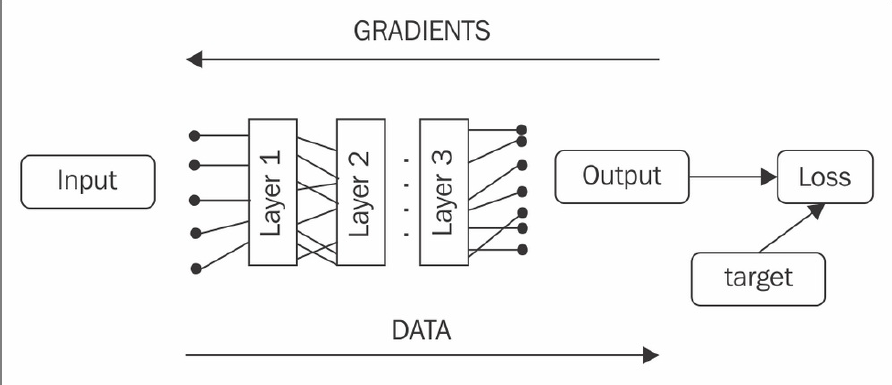• 静态图框架： Tensorflow1.0， Theano等框架。 在开始运算前， 你必须完整定义你的计算图（即输入到输出的具体结构）。 在开始运算前， 框架会对其进行计算优化。 静态图的优点是 运行效率更高速度更快， 缺点则是难以进行调试， 且无法在计算图生成后动态修改。
• 动态图框架： pytorch。 不需要预先定制好整个计算图。 框架会记录你当前的计算顺序， 储存对应的梯度值， 来服务于神经网络的计算。 调试 和 搭建网络的灵活性上， 动态图远远优于静态图。 这一方法也被称为 notebook gradient

Pytorch 的 张量， 内置了 梯度计算和跟踪机制， 因此， 你只需要把数据转换为张量， 然后使用pytorch提供的API进行操作即可。 每个张量都含有以下几个与梯度相关的属性：

• is_leaf: 如果张量由用户创建， 则为True； 有转化函数得到，则为False。

>>> v1 = torch.tensor([1.0, 1.0], requires_grad=True)
>>> v2 = torch.tensor([2.0, 2.0])
>>> v_sum = v1 + v2
>>> v_res = (v_sum*2).sum()
>>> v_res
tensor(12.)
>>> v1.is_leaf, v2.is_leaf
(True, True)
>>> v_sum.is_leaf, v_res.is_leaf
(False, False)
True
False
True
True
>>>
>>> v_res.backward()
tensor([ 2., 2.])
>>>


• 注意， 这里只能对v_res，即最后的结果使用backward()。 因为该方法只能对最后的标量结果使用， v_sum是张量， 无法使用backward()，去求出v1对于v_sum的梯度。

## Pytorch 的神经网络包：torch.nn

>>> import torch.nn as nn
>>> l = nn.Linear(2, 5) # 参数为 输入维度 和 输出维度
>>> v = torch.FloatTensor([1, 2])
>>> l(v)
tensor([ 0.1975, 0.1639, 1.1130, -0.2376, -0.7873])


• parameters(): 返回了一个迭代器， 里面包括所有需要求梯度的网络变量（比如网络的权重） 。 注：迭代器实现了__next__方法， 对迭代器a可以使用next(a)，获取a中的值。
• to(device)：使用GPU加速
• state_dict()：返回网络中的所有变量值

>>>l1 = nn.Linear(2,3)
>>>l2 = nn.Linear(2,3)
>>>a = l1.state_dict()
>>>a
Out:
OrderedDict([('weight',
tensor([[-0.3434,  0.6596],
[ 0.4947,  0.6010],
[-0.1376,  0.5829]])),
('bias', tensor([-0.2760,  0.4102,  0.4186]))])
>>>b = l2.state_dict()
>>>b
Out:
OrderedDict([('weight',
tensor([[ 0.2113,  0.0015],
[-0.4391,  0.2420],
[-0.2873,  0.0567]])),
('bias', tensor([0.6091, 0.2362, 0.2396]))])
Out: <All keys matched successfully>
>>>l2.state_dict()
Out:
OrderedDict([('weight',
tensor([[-0.3434,  0.6596],
[ 0.4947,  0.6010],
[-0.1376,  0.5829]])),
('bias', tensor([-0.2760,  0.4102,  0.4186]))])


>>> s = nn.Sequential(
... nn.Linear(2, 5),
... nn.ReLU(),
... nn.Linear(5, 20),
... nn.ReLU(),
... nn.Linear(20, 10),
... nn.Dropout(p=0.3),
... nn.Softmax(dim=1))
>>> s
Sequential (
(0): Linear (2 -> 5)
(1): ReLU ()
(2): Linear (5 -> 20)
(3): ReLU ()
(4): Linear (20 -> 10)
(5): Dropout (p = 0.3)
(6): Softmax ()
)
>>> s(torch.FloatTensor([[1,2]]))
tensor([[ 0.1410, 0.1380, 0.0591, 0.1091, 0.1395, 0.0635,
0.0607,
0.1033, 0.1397, 0.0460]])


nn.Linear, nn.ReLu, nn.Dropout, nn.Softmax，都是同名的知名网络层/激活函数的相应类。Sequential接受这些类作为参数， 并拼接成一个完整的神经网络。

## 自定义网络层

import torch
import torch.nn as nn

class OurModule(nn.Module):
def __init__(self, num_inputs, num_classes, dropout_prob=0.3):
super(OurModule, self).__init__()
self.pipe = nn.Sequential(
nn.Linear(num_inputs, 5),
nn.ReLU(),
nn.Linear(5, 20),
nn.ReLU(),
nn.Linear(20, num_classes),
nn.Dropout(p=dropout_prob),
nn.Softmax(dim=1)
)

def forward(self, x):
return self.pipe(x)

if __name__ == "__main__":
net = OurModule(num_inputs=2, num_classes=3)
print(net)
v = torch.FloatTensor([[2, 3]])
out = net(v)
print(out)


## 损失函数与优化器

### 损失函数

pytorch中，预定义了许多著名的损失函数：

• nn.MSELoss
• nn.BCELoss: Binary cross-entropy loss. 二元交叉熵。
• nn.CrossEntropyLoss: 用于多分类问题的交叉熵。

### 优化器

pytorch中，预定义了常用的优化器：

• torch.optim.SGD：随机梯度下降优化器
• torch.optim.RMSprop: RMSprop优化器

torch.optim.Adagrad(params, lr=0.01, lr_decay=0, weight_decay=0)

	for batch_samples, batch_labels in iterate_batches(data, batch_size=32):
batch_samples_t = torch.tensor(batch_samples))
batch_labels_t = torch.tensor(batch_labels))
out_t = net(batch_samples_t)
loss_t = loss_function(out_t, batch_labels_t)
loss_t.backward()
optimizer.step()


## 通过Tensorboard监控网络训练

• 损失值
• 在验证集和测试集上的表现
• 梯度 和 权重 的相关数据统计
• 学习率， 以及其他超参数

Tensorboard 就是 满足这一需求的经典工具。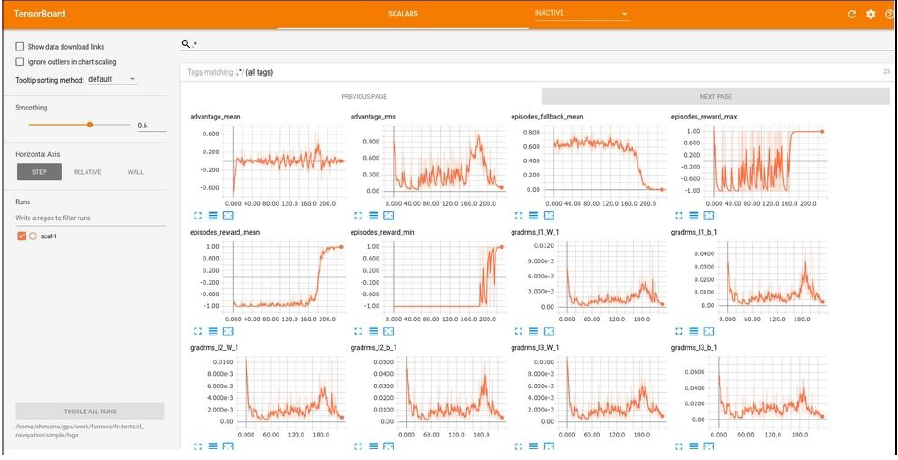• 进行训练， 并存储数据到本地字典
• 打开tensorboard的网络服务
• 打开浏览器， 输入正确的端口号， 观看监控结果
当你是使用非本地计算机时，这一功能尤其完美——你可以通过网页访问云端服务器的训练情况。

pip install tensorboard-pytorch下载相关的组件。下载该包会自动下载tensorboard。 接下来， 找到下载到的tensorboard.exe的路径， 将其添加到windows的环境变量中（这里不会的百度）。

import math
from tensorboardX import SummaryWriter

if __name__ == "__main__":
writer = SummaryWriter()

funcs = {"sin": math.sin, "cos": math.cos, "tan": math.tan}

for angle in range(-360, 360):
angle_rad = angle * math.pi / 180
for name, fun in funcs.items():

writer.close()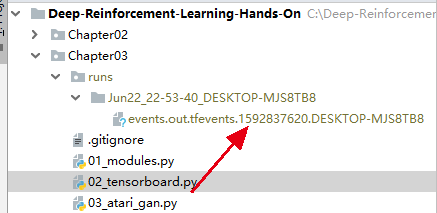tensorboard --logdir C:\Deep-Reinforcement-Learning-Hands-On\Chapter03\runs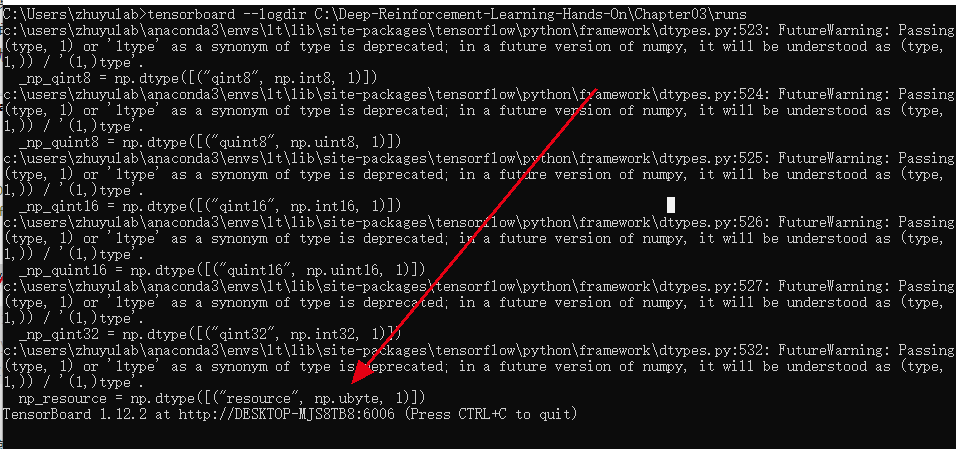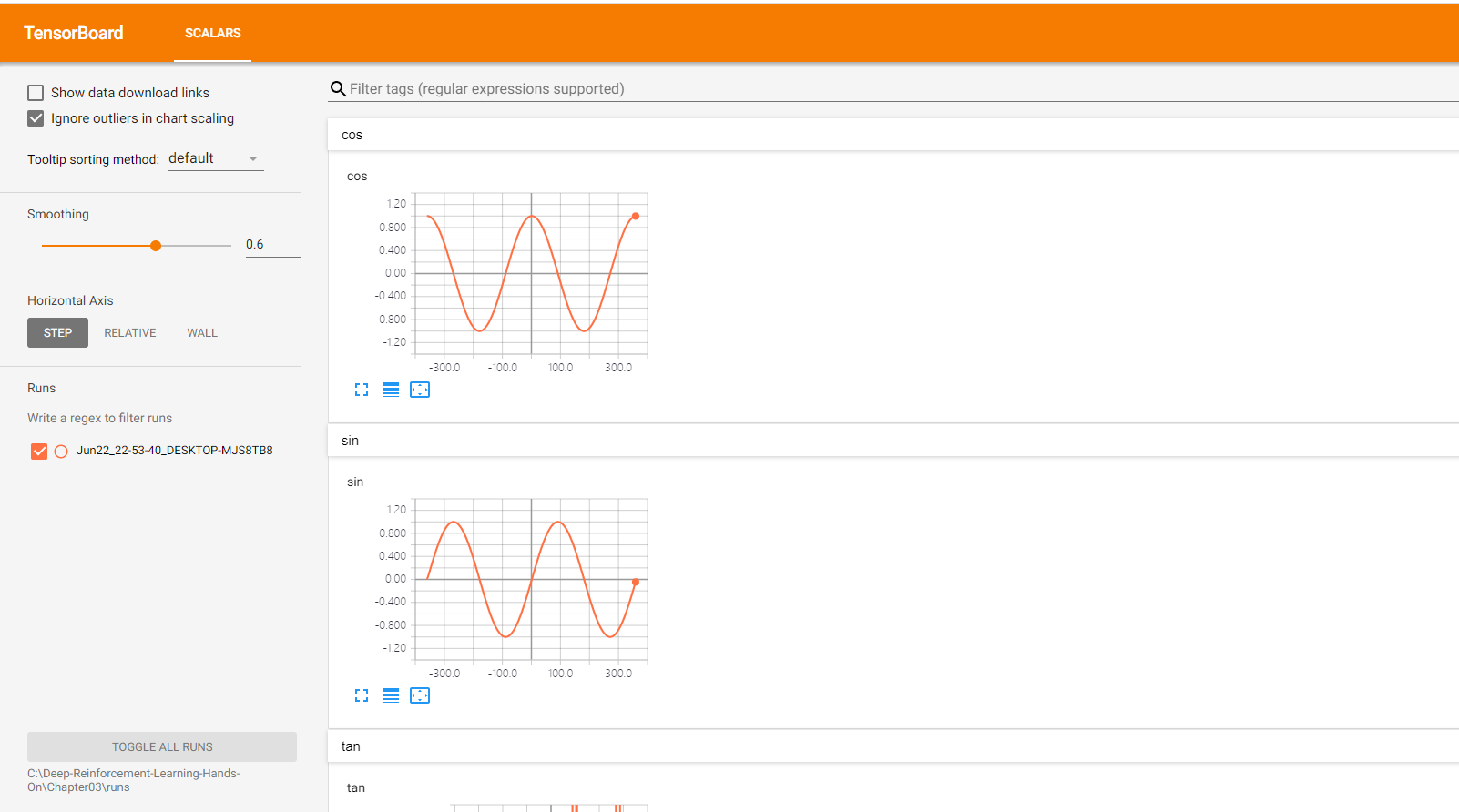09-171355

#### 17种深度强化学习算法用Pytorch实现（附链接）10-232892

#### 【代码集合】深度强化学习Pytorch实现集锦

07-222万+

#### pytorch使用记录（三） 多GPU训练

06-052876

#### 使用PyTorch，OpenAI Gym和Gazebo机器人模拟器为NVIDIA Jetson深度强化学习GPU库

05-265416

#### pytorch 深度学习之强化学习玩flappy bird

11-3014万+

#### 基于PyTorch的深度学习入门教程（一）——PyTorch安装和配置

07-051810

#### 用深度强化学习玩贪食蛇

04-21371

#### 深度强化学习——安装Windows10系统深度强化学习环境：Anaconda+PyTorch+Pycharm+Gym©️2020 CSDN 皮肤主题: 大白 设计师: CSDN官方博客点击重新获取扫码支付1.余额是钱包充值的虚拟货币，按照1:1的比例进行支付金额的抵扣。
2.余额无法直接购买下载，可以购买VIP、C币套餐、付费专栏及课程。余额充值